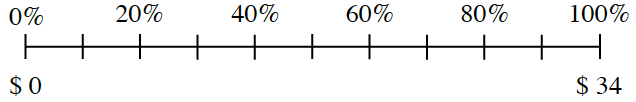Home > CC1MN > Chapter cc13 > Lesson cc13.1.2 > Problem3-33

3-33.

Maribel is taking advantage of the sale at Cassie’s Cashew Shoppe. She wants to figure out how much she will save on a purchase of $34$. Maribel’s percent ruler is shown below. Copy the ruler on your paper and help her figure out what $20\%$ of $34$ is. Homework Help ✎To start, it would be helpful to scale the lower portion of the number line. Do you remember how to do this?

Now that you know $10\%$ is equal to $3.40$, can you scale the rest of the number line to find the value of $20\%$?

First, we can find out how much money will be just one tick mark on the number line. Start by counting the number of tick marks ($10$). We know that $34$ will be distributed among these tick marks.

With the information gathered in Step 1, we can now find what amount will be labeled on the first tick mark ($10\%$). We will find this value by dividing the total $34$ ($100\%$) by the number of tick marks (10).

$\frac{34}{10}= \3.40$

Each tick mark represents $3.40$.